# RD Sharma Solutions Class 9 Chapter 16 Circles

Read RD Sharma Solutions Class 9 Chapter 16 Circles below, students should study RD Sharma class 9 Mathematics available on Studiestoday.com with solved questions and answers. These chapter wise answers for class 9 Mathematics have been prepared by teacher of Grade 9. These RD Sharma class 9 Solutions have been designed as per the latest NCERT syllabus for class 9 and if practiced thoroughly can help you to score good marks in standard 9 Mathematics class tests and examinations

Exercise 16.1

Question 1:  Fill in the blanks:
(i) All points lying inside/outside a circle are called ______ points/_______ points.
(ii) Circles having the same centre and different radii are called _____ circles.
(iii) A point whose distance from the centre of a circle is greater than its radius lies in _________ of the circle.
(iv) A continuous piece of a circle is _______ of the circle.
(v) The longest chord of a circle is a ____________ of the circle.
(vi) An arc is a __________ when its ends are the ends of a diameter.
(vii) Segment of a circle is a region between an arc and _______ of the circle.
(viii) A circle divides the plane, on which it lies, in _________ parts.
Solution:
(i) All points lying inside/outside a circle are called Interior points/ Exterior points.
(ii) Circles having the same centre and different radii are called Concentric circles.
(iii) A point whose distance from the centre of a circle is greater than its radius lies in the Exterior of the circle.
(iv) A continuous piece of a circle is Arc of the circle.
(v) The longest chord of a circle is a Diameter of the circle.
(vi) An arc is a Semi-circle when its ends are the ends of a diameter.
(vii) Segment of a circle is a region between an arc and centre of the circle.
(viii) A circle divides the plane, on which it lies, in three parts.

Question 2: Write the truth value (T/F) of the following with suitable reasons:
(i) A circle is a plane figure.
(ii) Line segment joining the center to any point on the circle is a radius of the circle,
(iii) If a circle is divided into three equal arcs each is a major arc.
(iv) A circle has only finite number of equal chords.
(v) A chord of a circle, which is twice as long as its radius is the diameter of the circle.
(vi) Sector is the region between the chord and its corresponding arc.
(vii) The degree measure of an arc is the complement of the central angle containing the arc.
(viii) The degree measure of a semi-circle is 1800.
Solution:
(i) True
(ii) True
(iii) True
(iv) False
(v) True
(vi) True
(vii) False
(viii) True
Exercise 16.2

Question 1: The radius of a circle is 8 cm and the length of one of its chords is 12 cm. Find the distance of the chord from the centre.
Solution: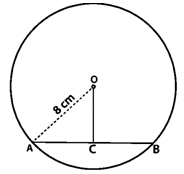It is given that, Radius of circle (OA) = 8 cm and Chord (AB) = 12cm
Draw a perpendicular OC on AB.
Perpendicular from centre to chord bisects the chord
AC = BC = 12/2
AC = BC = 6cm
ΔOCA is a right angled triangle
OA2 = AC2 + OC2 (Pythagoras theorem)
64 = 36 + OC2
OC2 = 64 – 36
OC2 = 28
OC = √28
OC = 5.291
Hence, the distance of the chord from the centre is 5.291cm.

Question 2: Find the length of a chord which is at a distance of 5 cm from the centre of a circle of radius 10 cm.
Solution: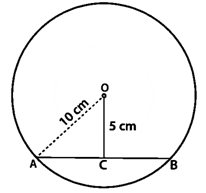It given that, distance of the chord from the centre = OC = 5 cm, Radius = OA = 10 cm
ΔOCA is a right angled triangle
OA2 = AC2 + OC2 (By Pythagoras theorem)
100 = AC2 + 25
AC2 = 100 – 25
AC2  = 75
AC = √75
AC = 8.66
OC is the Perpendicular on AB. So, the centre to chord bisects it.
AC = BC = 8.66cm
AB = AC + BC
AB = 8.66 + 8.66
AB = 17.32
Hence, the length of a chord AB is 17.32cm.

Question 3: Find the length of a chord which is at a distance of 4 cm from the centre of a circle of radius 6 cm.
Solution: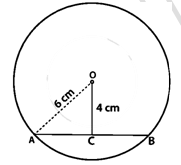It is given that,
Distance of the chord from the centre = OC = 4cm
ΔOCA is a right triangle
OA2 = AC2 + OC2 (By Pythagoras theorem)
36 = AC2 + 16
AC2 = 36 – 16
AC2 = 20
AC = √20
AC = 4.47cm
OC is the Perpendicular on AB. So, the centre to chord bisects it.
AC = BC = 4.47 cm
AB = AC + BC
AB = 4.47 + 4.47
AB = 8.94cm
Hence, the length of a chord is AB is 8.94cm.

Question 4: Two chords AB, CD of lengths 5 cm, 11 cm respectively of a circle are parallel. If the distance between AB and CD is 3 cm, find the radius of the circle.
Solution:It is given that,
AB = 5cm
CD = 11cm
PQ = 3cm
Perpendiculars OP on CD
Perpendiculars OQ on AB
OP = x cm and OC = OA = r cm
We know, perpendicular from centre to chord bisects it.
OP⊥CD, we have
CP = PD = 11/2 cm
OQ ⊥ AB
AQ = BQ = 5/2 cm
ΔOCP is a right triangle
OC2 = OP2 + CP2 (By Pythagoras theorem)
r2 = x2 + (11/2)2 ________(1)
ΔOQA is a right triangle
OA2= OQ2+ AQ2 (By Pythagoras theorem)
r2= (x-3)2 + (5/2)2 ________(2)
From equations (1) and (2), we get
(x-3)2 + (5/2)2 = x2 + (11/2)2(∴(a+b)2 = a2 + b2 + 2ab)
x2+6x+9+25/4 =x2+121/4
6x = 121/4 – 25/4 − 9
6x = 121/4 – 25/4 − 9/1
6x = (121 - 25 - 36)/4
6x = (121 - 25 - 36)/4
6x = 60/4
6x = 15
x = 15/6
x = 5/2
Put the value of x in equation (1),
r2 = (5/2)2 + (11/2)2
r = 25/4 + 121/4
r = 146/4
r = √146/4cm
Hence, the value of r is √146/4cm.

Question 5: Give a method to find the centre of a given circle.
Solution:
Steps of Construction:
1. Draw a circle with three points A, B, and C.
2. Becoming a member of AB and BC.
3. Draw perpendicular bisectors of chords AB and BC that cross at a point called O.
4. This point O is the circle's centre, so we know that the chord's perpendicular bisectors still move through it.Question 6: Prove that the line joining the mid-point of a chord to the centre of the circle passes through the mid-point of the corresponding minor arc.
Solution:It is given that, Let us C is the mid-point of chord AB.
In ΔOAC and ΔOBC
OA = OB (Radius of circle)
OC = OC (Common)
AC = BC (C is the mid-point of chord)
ΔOAC ≅ ΔOBC (by SSS condition)
∠AOC = ∠BOC (BY CPCT)
= mA ̅D ≅ mB ̅D
= A ̅D ≅ B ̅D
Therefore, D is the mid-point of arc AB.
Hence Proved

Question 7: Prove that a diameter of a circle which bisects a chord of the circle also bisects the angle subtended by the chord at the centre of the circle.
Solution: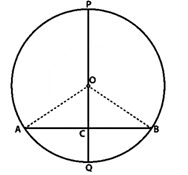It is given that, PQ is a diameter of circle which bisects the chord AB at C. (Given)
In ΔBOC and ΔAOC
OC = OC (Common)
AC = BC (Given)
ΔAOC ≅ ΔBOC (by SSS condition)
∠AOC = ∠BOC (CPCT)
PQ bisects ∠AOB
Hence proved

Question 8: Prove that two different circles cannot intersect each other at more than two points.
Solution:
A, B, and C are the points where two circle.
A, B, and C are then non-collinear, resulting in a single loop passing through all three points. This is in direct opposition to the fact that two defined circles move through A, B, and C.
As a result, no two circles can converge at more than two points.

Question 9:  A line segment AB is of length 5 cm. Draw a circle of radius 4 cm passing through A and B. Can you draw a circle of radius 2 cm passing through A and B? Give reason in support of your answer.
Solution: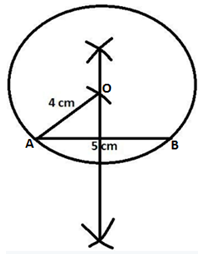(1) Draw a 5cm line section AB.

(2) Build AB's perpendicular bisectors.

(3) Draw an arc with a radius of 4cm that intersects the perpendicular bisector at point O. The required centre will be point O.

(4) Becoming a member of OA.

(5) Draw a circle with the centre O and the radius OA.

No, we can't draw a circle with a radius of 2 cm that passes between A and B because an arc with a radius of 2 cm and a centre of A would not intersect the perpendicular bisector, and we won't be able to locate the centre.

Question 10:  An equilateral triangle of side 9 cm is inscribed in a circle. Find the radius of the circle.

Solution: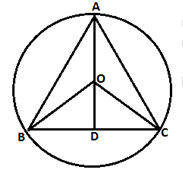AD is a perpendicular bisector of BC

BD = DC = 9/2cm.

O as circumcentre.

Circumcentre bisects bisector AD in ratio 2:1.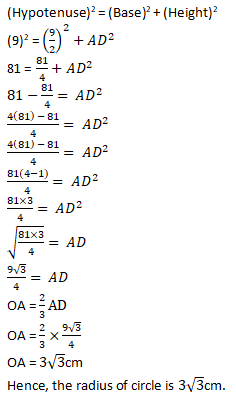Question 11:  Given an arc of a circle, complete the circle.
Solution:(1) Take three points on arc A, B, and C.

(2) Assemble with AB and BC.

(3) Draw the perpendicular bisectors of the chords AC and BC. The required centre of the required circle would then be O.

(4) Join OA.

(5) Complete the circle with the centre O and radius OA.

Question 12: Draw different pairs of circles. How many points does each pair have in common? What is the maximum number of common points?

Solution: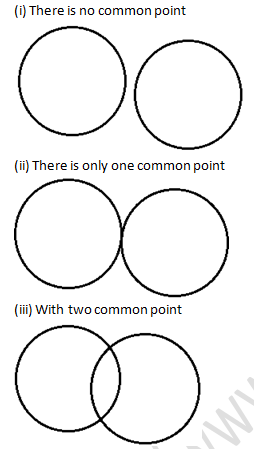Question 13: Suppose you are given a circle. Give a construction to find its centre.
Solution: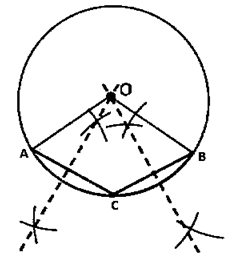(1) Take three points A, B and C on the given circle.

(2) Join AB and BC.

(3) Draw the perpendicular bisectors of chord AB and BC which intersect each other at O.

(4) Point O will be the required centre of the circle because we know that the perpendicular bisector of the chord always passes through the centre.

Question 14:  Two chords AB and CD of lengths 5 cm and 11 cm respectively of a circle are parallel to each other and are opposite side of its centre. If the distance between AB and CD is 6 cm, find the radius of the circle.

Solution:
Draw OM⊥AB and ON⊥CD.Join point O to B and D.
BM = AB/2 (Perpendicular from the centre bisects the chord)
BM = 5/2
ND = CD/2
ND = 11/2
Let us assumed that ON is x,
OM = 6 - x
In ΔMOB is a right triangle
OM2 + MB2 = OB2 (Pythagoras theorem)
(6 − x)2 + (5/2)2= OB2
36 + x2 − 12x + 25/4 = OB2 _______(i)
In ΔNOD is a right triangle,
ON2 + ND2 = OD2 (Pythagoras theorem)
x2 + (11/2)2= OD2
x2 + 121/4 = OD2_______ (ii)
OB = OD (Radii of a circle)
From equation (i) and (ii) we get,
OB2 = OD2
36 + x2 − 12x + 25/4 = x2 + 121/2
x2 − 12x – x2  = 121/4-25/4-36
− 12x = (121-25-144)/4
− 12x = -48/4
12x = 12
x = 12/12
x = 1
Value of x put in equation (ii)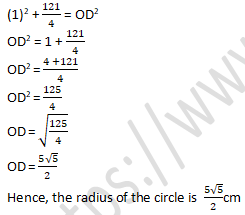Question 15:  The lengths of two parallel chords of a circle are 6 cm and 8 cm. If the smaller chord is at a distance of 4 cm from the centre, what is the distance of the other chord from the centre?
Solution:Distance of smaller chord AB from centre of circle = 4 cm, OM = 4 cm
MB = AB/2
MB = 6/2
MB = 3cm
ΔOMB is a right triangle
OB2 = OM2 + MB2 (Pythagoras theorem)
OB2 = (4)2 + (3)2
OB2 = 16 + 9
OB = √25
OB = 5 cm

OD = OB = 5 cm [Radii of a circle]
ND = CD/2
ND = 8/2
ND = 4cm

In ΔOND is a right triangle
OD2 = ON2 + ND2 (Pythagoras theorem)
(5)2 = ON2 + (4)2
25 - 16 = ON2
√9 = ON
3 = ON
ON = 3cm
Hence, the distance of bigger chord from the circle is 3cm.

Exercise 16.3

Question 1: Three girls Ishita, Isha and Nisha are playing a game by standing on a circle of radius 20 m drawn in a park. Ishita throws a ball to Isha, Isha to Nisha and Nisha to Ishita. If the distance between Ishita and Isha and between Isha and Nisha is 24 m each, what is the distance between Ishita and Nisha.
Solution:Let R, S and M be the position of Ishita, Isha and Nisha respectively.

AR = AS = 24/2

AR = AS = 12cm

It is given that, Radius of circle = OR = OS = OM = 20cm

ΔOAR is a right angle,

OR= OA2+ AR2   (Pythagoras theorem)

(20)= OA2+(12)

400=OA2+144

-OA= 144-400

-OA2=-256

OA=√256

OA=16m _________(i)

OABC is a kite since OA = OC and AB = BC

In ΔRSM,

∠RCS = 90° and RC = CM _________(ii)

Now, Area of ΔORS = Area of ΔORS

1/2 × OA × RS = 1/2 × RC × OS

OA × RS = RC × OS

384 = RC × 20

384/20 = RC

19.2 = RC

RC = 19.2m

RC = CM

RM = 2(19.2)

RM = 38.4

Hence, the distance between Ishita and Nisha is 38.4m.

Question 2:  A circular park of radius 40 m is situated in a colony. Three boys Ankur, Amit and Anand are sitting at equal distance on its boundary each having a toy telephone in his hands to talk to each other. Find the length of the string of each phone.

Solution:Since, AB = BC = CA. So, ABC is an equilateral triangle

Radius = OA = 40 m (Given)

We know, medians of equilateral triangle pass through the circumcentre and intersect each other at the ratio 2 : 1.

Here AD is the median of equilateral triangle ABC, we can write:

OA/OD = 2/1

or 40/OD = 2/1

or OD = 20 m

Therefore, AD = OA + OD = (40 + 20) m = 60 m

ΔADC is a right angle triangle

AC2 = AD2 + DC2 (By Pythagoras theorem)

AC= 602+  (AC/2)2

AC2- AC2/4  =3600

3/4 AC2 = 3600

AC2 = (3600 × 4)/3

AC2 = 4800

AC = 40√3m

Hence, the length of string will be 40√3m.

Exercise 16.4

Question 1: In figure, O is the centre of the circle. If ∠APB = 50°, find ∠AOB and ∠OAB.Solution:
It is given that, ∠APB = 50°
∠AOB = 2∠APB (Degree measure theorem)
∠AOB = 2 × 50°
∠AOB = 100°
OA = OB (Radius of circle)
∠OAB = ∠OBA (Angles opposite to equal sides)
Let us assumed that, ∠OAB = m
In ΔOAB,
∠OAB + ∠OBA + ∠AOB = 180° (Angle sum property)
m + m + 100° = 180°
2m = 180° – 100°
2m = 80°
m = (80°)/2
m = 40°
∠OAB = ∠OBA = 40°

Question 2: In figure, it is given that O is the centre of the circle and ∠AOC = 150°. Find ∠ABC.Solution:
It is given that, ∠AOC = 150°
∠ABC = ((reflex ∠AOC))/2 _________(1) (By degree measure theorem)
∠AOC + reflex ∠AOC = 360° (Complex angle)
150° + reflex ∠AOC = 360°
reflex ∠AOC = 360°−150°
reflex ∠AOC = 210°
∠ABC = (210°)/2
∠ABC = 105°

Question 3: In figure, O is the centre of the circle. Find ∠BAC.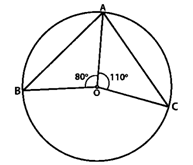Solution:
It is given that, ∠AOB = 80° and ∠AOC = 110°
We know that,
∠AOB + ∠AOC + ∠BOC = 360°
80° + 110° + ∠BOC = 360°
190° + ∠BOC = 360°
∠BOC = 360° – 190°
∠BOC = 170°

∠BOC = 2∠BAC (Degree measure theorem)
170° = 2∠BAC
(170°)/2 = ∠BAC
85° = ∠BAC
∠BAC = 85°

Question 4: If O is the centre of the circle, find the value of x in each of the following figures.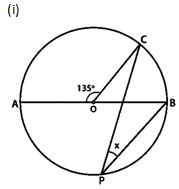Solution:
It is given that, ∠AOC = 135°
∠AOC + ∠BOC = 180° (Linear pair of angles)
135° +∠BOC = 180°
∠BOC = 180°−135°
∠BOC = 45°
∠BOC = 2∠CPB (By degree measure theorem)
45° = 2x
(45°)/2 = x
x = 22.5°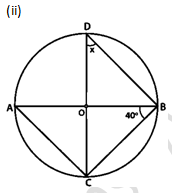Solution:
It is given that, ∠ABC=40°
∠ACB = 90° (Angle in semicircle)
In ΔABC,
∠CAB + ∠ACB + ∠ABC = 180° (angle sum property)
∠CAB + 90° + 40° = 180°
∠CAB = 180°− 90°− 40°
∠CAB = 180°− 130°
∠CAB = 50°
∠CDB = ∠CAB (Angle is on same segment)
x = 50°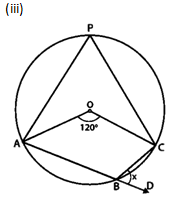Solution:
It is given that, ∠AOC = 120°
∠AOC = 2∠APC (Degree measure theorem)
120° = 2∠APC
∠APC = (120°)/2
∠APC = 60°

∠APC + ∠ABC = 180° (Sum of opposite angles of cyclic quadrilaterals is180°)
60° + ∠ABC=180°
∠ABC = 180°− 60°
∠ABC = 120°

∠ABC + ∠DBC = 180° [Linear pair of angles]
120° + x = 180°
x = 180°− 120°
x = 60°
Hence, the value of x is 60°.Solution:
It is given that, ∠CBD = 65°
∠ABC + ∠CBD = 180° (Linear pair)
∠ABC + 65° = 180°
∠ABC = 180°− 65°
∠ABC = 115°
Reflex ∠AOC = 2∠ABC (Degree measure theorem)
x = 2(115°)
x = 230°
Hence, the value of x is 230°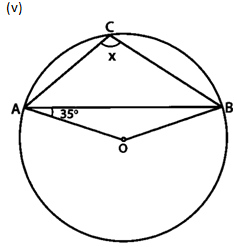Solution:
It is given that, ∠OAB = 35°
∠OBA = ∠OAB = 35° (angles opposite to equal radii)
In ΔAOB
∠AOB + ∠OAB + ∠OBA = 180° (angle sum property)
∠AOB + 35° + 35° = 180°
∠AOB + 70° = 180°
∠AOB = 180° – 70°
∠AOB = 110°
∠AOB + reflex∠AOB = 360° (Complex angle)
Put the value of ∠AOB
110° + reflex∠AOB = 360°
reflex∠AOB = 360° – 110°
reflex∠AOB = 250°
reflex ∠AOB = 2∠ACB (Degree measure theorem)
250° = 2x
x = (250°)/2
x = 125°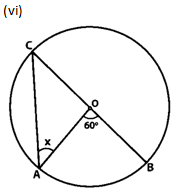Solution:
It is given that, ∠AOB = 60°
reflex∠AOB = 2∠OAC (degree measure theorem)
60° = 2∠ OAC
∠OAC = (60°)/2
∠OAC = 30° (Angles opposite to equal radius)
x = 30°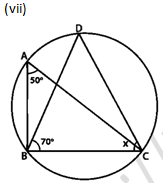Solution:
It is given that, ∠BAC = 50° and ∠DBC = 70°
∠BDC = ∠BAC = 50° (Angle on same segment)
In ΔBDC
∠BDC + ∠BCD + ∠DBC = 180° (angle sum property)
50° + x + 70° = 180°
x = 180°− 50°− 70°
x = 60°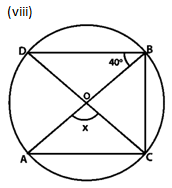Solution:
It is given that, ∠DBO = 40°
∠DBC = 90° (Angle in a semicircle)
∠DBO + ∠OBC = 90°
40° + ∠OBC = 90°
∠OBC = 90°− 40°
∠OBC = 50°
∠AOC = 2∠OBC (By degree measure theorem)
x = 2 × 50°
x = 100°Solution:
It is given that, ∠CAD = 28, ∠ADB = 32 and ∠ABC = 50
In ΔDAB:
∠ADB + ∠DAB + ∠ABD = 180° (Angle sum property)
32° + ∠DAB + 50° = 180°
82° + ∠DAB = 180°
∠DAB = 180°− 82°
∠DAB = 98°
∠DAB + ∠DCB = 180° (the sum of opposite angles of cyclic quadrilateral is 180°)
98° + x = 180°
x = 180°− 98°
x = 82°
Hence, the value x is 82°.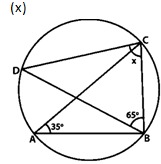Solution:
It is given that, ∠BAC = 35° and ∠DBC = 65°
∠BDC = ∠BAC = 35° (Angle in same segment)
In ΔBCD
∠BDC + ∠BCD + ∠DBC = 180° (Angle sum property)
35° + x + 65° = 180°
100° + x = 180°
x = 180° – 100°
x = 80°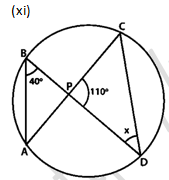Solution:
It is given that, ∠ABD = 40°, ∠CPD = 110°
∠ACD = ∠ABD = 40° (Angle in same segment)
In ΔPCD
∠PCD + ∠CPO + ∠PDC = 180° (Angle sum property)
40° + 110° + x = 180°
150° + x = 180°
x = 180° − 150°
x = 30°
Hence, the value of x is 30 degrees.Solution:
It is given that, ∠BAC = 52°
∠BDC = ∠BAC = 52° (Angle in same segment)
OD = OC (radius of a circle)
∠ODC = ∠OCD (Opposite angle to equal radius)
x = 52°
Hence, the measure of ∠x is 52°.

Question 5: O is the circumcentre of the triangle ABC and OD is perpendicular on BC. Prove that ∠BOD = ∠A.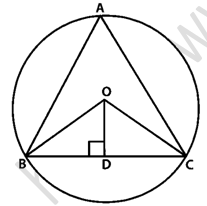Solution:
In ΔOBD and ΔOCD

OB = OC (Radius of a circle)

OD = OD (Common)

∠ODB = ∠ODC (Both 90°)

ΔOBD ≅ ΔOCD (By RHS Condition)

∠BOD = ∠COD (CPCT)

∠BOC = 2∠BAC (Degree measure theorem)

2∠BOD = 2∠BAC

∠BOD = ∠BAC

Hence proved

Question 6: In figure, O is the centre of the circle, BO is the bisector of ∠ABC. Show that AB = AC.Solution:
It is given that, BO is the bisector of ∠ABC, then,

∠ABO = ∠CBO _________(i)

OB = OA = OB = OC (Radius of circle)

∠OAB = ∠OCB (opposite angles to equal sides) _________(ii)

∠ABO = ∠DAB (opposite angles to equal sides) _________(iii)

From equations (i), (ii) and (iii), we get

∠OAB = ∠OCB

In ΔOAB and ΔOCB

∠OAB = ∠OCB (Proved above)

∠OBA = ∠OBC (given)

OB = OB (Common)

ΔOAB ≅ ΔOCB (By AAS condition)

AB = BC (By CPCT)

Question 7: In figure, O is the centre of the circle, then prove that ∠x = ∠y + ∠z.Solution:
From the given figure we conclude that,

∠3 = ∠4 (Angles in same segment) ______(i)

∠x = 2∠3 (By degree measure theorem)

∠x = ∠3 + ∠3

∠x = ∠3 + ∠4

∠y = ∠3 + ∠1 (Sum of interior angles are equal to exterior angle) ____________(ii)

∠3 = ∠y − ∠1 _________(iii)

∠4 = ∠z + ∠1 _________(iv) (By exterior angle property)

Now, from equations (ii), (iii) and (iv), we get

∠x = ∠y − ∠1 + ∠z + ∠1

∠x = ∠y + ∠z

Hence proved

Exercise 16.5

Question 1: In figure, ΔABC is an equilateral triangle. Find m∠BEC.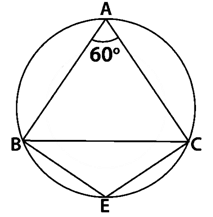Solution:
It is given that, ΔABC is an equilateral triangle
∠BAC + ∠BEC = 180° (Opposite angles of quadrilateral)
60° + ∠BEC = 180° (each angle of equilateral triangle is 60°)
∠BEC = 180° – 60°
∠BEC = 120°

Question 2: In figure, Δ PQR is an isosceles triangle with PQ = PR and m∠PQR=35°. Find m∠QSR and m∠QTR.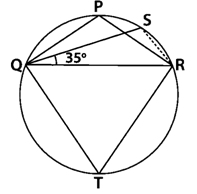Solution:
It is given that, ΔPQR is an isosceles triangle.

PQ = PR

m∠PQR = 35°

In ΔPQR

∠PQR = ∠PRQ = 35° (Angle opposite to equal sides)

∠P + ∠Q + ∠R = 180° (angle sum property)

∠P + 35° + 35° = 180°

∠P + 70° = 180°

∠P = 180° – 70°

∠P = 110°

∠QSR + ∠QTR = 180° (Opposite angles of quadrilateral)

110° + ∠QTR = 180°

∠QTR = 180° - 110°

∠QTR = 70°

Hence, the measure of ∠QTR is 70°.

Question 3: In figure, O is the centre of the circle. If ∠BOD = 160°, find the values of x and y.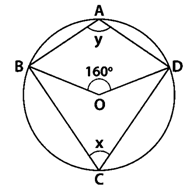Solution:
It is given that, ∠BOD is 160°.

∠BOD = 2∠BCD (Degree measure theorem)

160° = 2x

(160°)/2 = x

80° = x

x = 80°

y + x = 180°_______(i)

Putting value of x in equation (i)

y + 80° = 180°

y = 100°

Hence, the value of x is 80° and y is 100°.

Question 4: In figure, ABCD is a cyclic quadrilateral. If ∠BCD = 100° and ∠ABD = 70°, find ∠ADB.Solution:
It is given that, ABCD is cyclic quadrilateral
∠ADB + ∠DAB + ∠ABD = 180° (Angle sum property)
∠ADB + 80° + 70° = 180°

Question 5: If ABCD is a cyclic quadrilateral in which AD||BC (figure). Prove that ∠B = ∠C.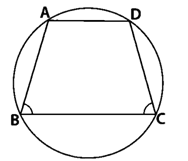Solution:
It is given that, ABCD is a cyclic quadrilateral with AD ll BC

∠A + ∠C = 180° ________(1) (Opposite angles of cyclic quadrilateral)

∠A + ∠B = 180° ________(2) (Co-interior angles)

Form equation (1) and (2), we get

∠B = ∠C

Hence proved

Question 6: In figure, O is the centre of the circle. Find ∠CBD.Solution:
It is given that, ∠BOC is 100°.

∠AOC = 2∠APC (Degree measure theorem)

100° = 2∠APC

(100°)/2 = ∠APC

∠APC = 50°

∠APC + ∠ABC = 180° (Opposite angles of a cyclic quadrilateral)

50° + ∠ABC = 180°

∠ABC = 180° - 50°

∠ABC = 130°

∠ABC + ∠CBD = 180° (Linear pair)

130° + ∠CBD = 180°

∠CBD = 180° - 130°

∠CBD = 50°

Hence, the measure of ∠CBD is 50°.

Question 7:  In figure, AB and CD are diameters of a circle with centre O. If ∠OBD = 500, find ∠AOC.Solution:
It is given that ∠OBD is 50°.
AB and CD are the diameters of the circles with centre O.
∠DBC = 90° _______(i) (Angle in the semi-circle)
∠DBC = 50° + ∠OBC
90° = 50° + ∠OBC
90°- 50° = ∠OBC
40° = ∠OBC
∠OBC = 40°
∠AOC = 2 ∠ABC (degree measure theorem)
∠AOC = 2∠OBC
∠AOC = 2 x 40°
∠AOC = 80°

Question 8: On a semi-circle with AB as diameter, a point C is taken, so that m(∠CAB) = 30°. Find m(∠ACB) and m(∠ABC).
Solution:
It is given that, m(∠CAB) = 30°
We know that angle in a semi-circle is a right angle.
∠ACB = 90°
In △ABC,
∠CAB + ∠ACB + ∠ABC = 180° (angle sum property)
30° + 90° + ∠ABC = 180°
120° + ∠ABC = 180°
∠ABC = 180°- 120°
∠ABC = 60°
Hence, the measure of ∠ACB is 90° and ∠ABC is 60°.

Question 9: In a cyclic quadrilateral ABCD if AB||CD and ∠B = 70° , find the remaining angles.
Solution:
It is given that, a cyclic quadrilateral ABCD with AB||CD and ∠B = 70°.
∠B + ∠C = 180° (Co-interior angle)
70° + ∠C = 180°
∠C = 180°- 70°
∠C = 110°

∠B + ∠D = 180° (Opposite angles of cyclic quadrilateral)
70° + ∠D = 180°
∠D = 180° - 70°
∠D = 110°

∠A + ∠C = 180° (Opposite angles of cyclic quadrilateral)
∠A + 110° = 180°
∠A + 110° = 180°- 110°
∠A = 70°
Hence, the measure of ∠A is 70° , ∠C is 110° and ∠D is 110°

Question 10: In a cyclic quadrilateral ABCD, if m ∠A = 3(m∠C). Find m∠A.
Solution:
We know that,
∠A + ∠C = 180° _______(1) (Opposite angles of cyclic quadrilateral)
It is given that, m∠A = 3(m∠C)
∠A = 3∠C ______(2)
Put the value of ∠A in equation (1)
3∠C + ∠C = 180°
4∠C = 180°
∠C = 45°
Put the value of ∠C in equation (2)
∠A = 3 × 45°
∠A = 135°

Question 11: In figure, O is the centre of the circle ∠DAB = 50°. Calculate the values of x and y.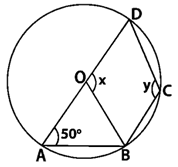Solution:
It is given that, ∠DAB is 50°

We know that,

x = 2(50°)

x = 100°

From the figure we know that, ABCD is a cyclic quadrilateral

∠A + ∠C = 180°

50° + y = 180°

y = 180°- 50°

y = 130°

Hence, the measure of angle x and y is 100° and 130° respectively.

Exercise VSAQs .......................

Question 1: In figure, two circles intersect at A and B. The centre of the smaller circle is O and it lies on the circumference of the larger circle. If ∠APB = 70°, find ∠ACB.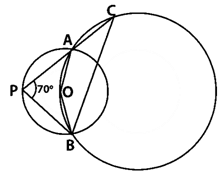Solution:
∠AOB = 2 ∠APB (Degree measure theorem)
∠AOB = 2 × 70°
∠AOB = 140°
∠ACB + ∠AOB = 180°
∠ACB + 140° = 180°
∠ACB = 40°

Question 2: In figure, two congruent circles with centres O and O’ intersect at A and B. If ∠AO’B = 50°, then find ∠APB.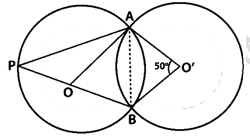Solution:
∠AOB = ∠AO’B = 50°
∠APB = (∠AOB)/2 (By degree measure theorem)
∠APB = (50°)/2
∠APB = 25°

Question 3: In figure, ABCD is a cyclic quadrilateral in which ∠BAD=75°, ∠ABD=58° and ∠ADC=77°, AC and BD intersect at P. Then, find ∠DPC.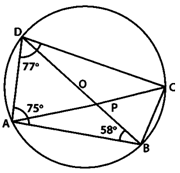Solution:
It is given that, ABCD is a cyclic quadrilateral
∠DBA = ∠DCA = 58°___________(1) (Angles in same segment)
∠A +∠C = 180° (Sum of opposite angles is 180 degrees)
75° + ∠C = 180°
∠C = 180° - 75°
∠C = 105°

∠ACB + ∠ACD = 105°
∠ACB + 58° = 105°
∠ACB = 47° …(2)

∠ACB = ∠ADB = 47°(Angles in same segment)
It is given ∠D = 77°
∠BDC + 47° = 77°
∠BDC = 30°

In ∆DPC
∠PDC + ∠DCP + ∠DPC = 180°
30° + 58° + ∠DPC = 180°
88° + ∠DPC = 180°
∠DPC = 180° - 88°
∠DPC = 92°

Question 4: In figure, if ∠AOB = 80° and ∠ABC=30°, then find ∠CAO.Solution:
It is given that, ∠AOB = 80° and ∠ABC = 30°
Join OC
Central angle subtended by arc AC = ∠COA
∠COA = 2 x ∠ABC
∠COA = 2 x 30°
∠COA = 60°
In ∆OCA,